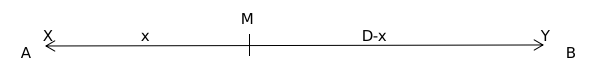Question 49

# Trains A and B start traveling at the same time towards each other with constant speeds from stations X and Y, respectively. Train A reaches station Y in 10 minutes while train B takes 9 minutes to reach station X after meeting train A. Then the total time taken, in minutes, by train B to travel from station Y to station X is

SolutionM - First meeting point

Let the speeds of trains A and B be 'a' and 'b', respectively.

$$\frac{x}{a}=\ \frac{\ D-x}{b}$$

It is given,

$$\frac{D}{a}=10$$ and $$\frac{x}{b}=9$$

$$\frac{x}{\frac{D}{10}}=\ \frac{\ D-x}{\frac{x}{9}}$$

$$\frac{10x}{D}=\ \frac{\ 9D-9x}{x}$$

$$10x^2=\ \ 9D^2-9Dx$$

$$10x^2+9Dx-9D^2=\ 0$$

Solving, we get $$x=\frac{3D}{5}$$

$$\frac{x}{b}=9$$

$$\frac{3D}{b\times5}=9$$

$$\frac{D}{b}=15$$

The total time taken by train B to travel from station Y to station X is 15 minutes.

### View Video Solution

• All Quant CAT Formulas and shortcuts PDF
• 30+ CAT previous papers with solutions PDF

##### SHIVA PENDEM

2 days, 6 hours ago

only video explanation was excellent

##### Parul Singh

3 months, 1 week ago

how (P+15) (P-6), I understood the formula (a+b) (a-b) but how 15 and 6

##### sandeep sandy

1 month, 2 weeks ago

(p+15) = 0 and (p-6) = 0 sp p = -15 and p = 6

##### keshab giri

3 months, 2 weeks ago

Thank you

##### Dishant Makwana

3 months, 2 weeks ago

not un
derstand

##### Aman Lalwani

9 months ago

Not clearly explaining the ratio portion after they met

OR